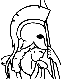#Introduction to Linear Optimization Table of Contents:

1. Introduction

1. Variants of the linear programming problem
2. Examples of linear programming problems
3. Piecewise linear convex objective functions
4. Graphical representation and solution
5. Linear algebra background and notation
6. Algorithms and operation counts
7. Exercises
8. History, notes, notes and sources

2. The geometry of linear programming

1. Polyhedra and convex sets
2. Extreme points, vertices, and basic feasible solutions
3. Polyhedra in standard form
4. Degeneracy
5. Existence of extreme points
6. Optimality of extreme points
7. Representation of bounded polyhedra
8. Projections of polyhedra: Fourier-Motzkin elimination
9. Summary
10. Exercises
11. Notes and sources

3. The simplex method

1. Optimality conditions
2. Development of the simplex method
3. Implementations of the simplex method
4. Anticycling: lexicography and Bland's rule
5. Finding an initial basic feasible solution
6. Column geometry and the simplex method
7. Computational efficiency of the simplex method
8. Summary
9. Exercises
10. Notes and sources

4. Duality theory

1. Motivation
2. The dual problem
3. The duality theorem
4. Optimal dual variables as marginal costs
5. Standard form problems and the dual simplex method
6. Farkas' lemma and linear inequalities
7. From separating hyperplanes to duality
8. Cones and extreme rays
9. Representation of polyhedra
10. General linear programming duality
11. Summary
12. Exercises
13. Notes and sources

5. Sensitivity analysis

1. Local sensitivity analysis
2. Global dependence on the right-hand side vector
3. The set of all dual optimal solutions
4. Global dependence on the cost vector
5. Parametric programming
6. Summary
7. Exercises
8. Notes and sources

6. Large scale optimization

1. Delayed column generation
2. The cutting stock problem
3. Cutting plane methods
4. Dantzig-Wolfe decomposition
5. Stochastic programming and Benders decomposition
6. Summary
7. Exercises
8. Notes and sources

7. Network flow problems

1. Graphs
2. Formulation of the network flow problem
3. The network simplex algorithm
4. The negative cost cycle algorithm
5. The maximum flow problem
6. Duality in network flow problems
7. Dual ascent methods
8. The assignment problem and the auction algorithm
9. The shortest path problem
10. The minimum spanning tree problem
11. Summary
12. Exercises
13. Notes and sources

8. Complexity of linear programming and the ellipsoid method

1. Efficient algorithms and computational complexity
2. The key geometric result behind the ellipsoid method
3. The ellipsoid method for the feasibility problem
4. The ellipsoid method for optimization
5. Problems with exponentially many constraints
6. Summary
7. Exercises
8. Notes and sources

9. Interior point methods

1. The affine scaling algorithm
2. Convergence of affine scaling
3. The potential reduction algorithm
4. The primal path following algorithm
5. The primal-dual path following algorithm
6. An overview
7. Exercises
8. Notes and sources

10. Integer programming formulations

1. Modeling techniques
2. Guidelines for strong formulations
3. Modeling with exponentially many constraints
4. Summary
5. Exercises
6. Notes and sources

11. Integer programming methods

1. Cutting plane methods
2. Branch and bound
3. Dynamic programming
4. Integer programming duality
5. Approximation algorithms
6. Local search
7. Simulated annealing
8. Summary
9. Exercises
10. Notes and sources

12. The art in linear optimization

1. Modeling languages for linear optimization
2. Optimization libraries and general observations
3. The fleet assignment problem
4. The air traffic flow management problem
5. The job shop scheduling problem
6. Summary
7. Exercises
8. Notes and sources

References

Index